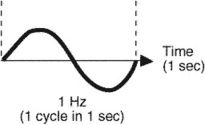# hertz

(redirected from Centihertz)
Also found in: Dictionary, Thesaurus, Medical.

## hertz

(hûrts) [for Heinrich R. HertzHertz, Heinrich Rudolf
, 1857–94, German physicist. He confirmed J. C. Maxwell's electromagnetic theory and in the course of experiments (1886–89) produced and studied electromagnetic waves (known also as hertzian waves, or radio waves).
], abbr. Hz, unit of frequency, equal to 1 cycle per second. The term is combined with metric prefixes to denote multiple units such as the kilohertz (1,000 Hz), megahertz (1,000,000 Hz), and gigahertz (1,000,000,000 Hz).

## hertz

(herts) Symbol: Hz. The SI unit of frequency, defined as the frequency of a periodic phenomenon that has a period of one second. The frequency range of electromagnetic radiation is about 3000 Hz (very low frequency radio waves) to about 1022 Hz (high-frequency gamma rays).

## hertz

[hərts]
(physics)
Unit of frequency; a periodic oscillation has a frequency of n hertz if in 1 second it goes through n cycles. Also known as cycle per second (cps). Symbolized Hz.

## hertz

A unit of frequency, abbr. Hz; one cycle per second.

## hertzThe frequency of any cyclic repetition. One hertz (Hz) is one cycle per second. The number of cycles per second is expressed in hertz. Kilohertz (kHz) is a frequency of one thousand cycles per second. Megahertz (MHz) is a frequency of one million cycles per second. The term is named after Heinrich Hertz, a German physicist (1857–1894). Also called a cycle.

## hertz

the derived SI unit of frequency; the frequency of a periodic phenomenon that has a periodic time of 1 second; 1 cycle per second.

## Hertz

1. Gustav . 1887--1975, German atomic physicist. He provided evidence for the quantum theory by his research with Franck on the effects produced by bombarding atoms with electrons: they shared the Nobel prize for physics (1925)
2. Heinrich Rudolph . 1857--94, German physicist. He was the first to produce electromagnetic waves artificially

## Hertz

Abbreviated "Hz," one Hertz is equal to one cycle per second. In 1883, Heinrich Hertz detected electromagnetic waves, and his name was adopted to measure the number of electromagnetic waves, or cycles, in a signal. Hertz is widely used to refer to the clock rate of a CPU; for example, 2 GHz means two billion cycles per second. The term is also used for other repeating cycles such as frame rate; for example, a 60 Hz TV displays 60 frames per second. See MHz, GHz and space/time.
Site: Follow: Share:
Open / Close